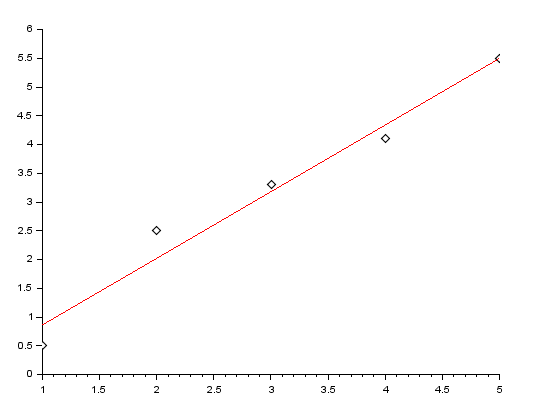# Linear Fitting – SCILAB

I have written a code that will calculate the equation of the best fit-line for a given set of data-points.

The procedure is based on least square approximation, which, in simple words,works by finding a line that is at a minimum distance possible from all the points.

Let’s say you have the x-axis points stored in a matrix, ‘x’ & the y-axis points stored in a matrix ‘y’. Then the following code returns the value of the slope, ‘m’ and intercept ‘c’ of the best fit-line, and also the fitted points which are basically the points of the y-axis obtained from the equation of the best fit-line.

CODE:

```//Linear Fit
//To fit a given set of data-points to a line.
//Written By: Manas Sharma(www.bragitoff.com)
funcprot(0);
function [f,m,c]=linefit(x,y)
n=size(x);
if n(2)<n(1) then
n=n(2)
else
n=n(1);
end
xsum=0;
ysum=0;
xysum=0;
x2sum=0;
for i=1:n
xsum=x(i)+xsum;
ysum=y(i)+ysum;
x2sum=x(i)*x(i)+x2sum;
xysum=x(i)*y(i)+xysum;
end
m=(n*xysum-xsum*ysum)/(n*x2sum-xsum*xsum);
c=(x2sum*ysum-xsum*xysum)/(x2sum*n-xsum*xsum);
for i=1:n
f(i)=m*x(i)+c;
end
endfunction

```

Sample Demo:

```x=[1,2,3,4,5];
y=[2,4,6,8,10];
[yfit,m,c]=linefit(x,y)

```

Output:
c =

0.
m =

2.
yfit =

2.
4.
6.
8.
10.

In this example the y-axis points were simply twice of the value of x-axis points. Hence the equation of line, we get is  y=mx+c=>y=2x.

Now let’s try something not so straight forward.

Sample Demo:

```x=[1,2,3,4,5];
y=[0.5,2.5,3.3,4.1,5.5];
[yfit,m,c]=linefit(x,y)

```

Output:

c  =

• 0.3
m  =

1.16
yfit  =

0.86
2.02
3.18
4.34
5.5

We can now even plot the observed data-points and the fitted points(yfit) and compare the two.

```plot2d(x,y,-5)  //dotted points for the original/observed data-points
plot2d(x,yfit,5) //red line for the fitted points

```

Output:I have created a module in SCILAB which contains the above macro, and once installed can be used as an in-built function. You can download it from here: https://atoms.scilab.org/toolboxes/curvefit

Leave your questions/suggestion/corrections in the comments section down below and I’ll get back to you soon.[wpedon id="7041" align="center"]

## 6 thoughts on “Linear Fitting – SCILAB”

1. Scilab says that there’s an error in the 3rd line. Some operator is missing. Idk…

1. I noticed that the if condition has gotten formatted wrongly due to some HTML problem.
Did you try changing this: “

```if n(2)>n(1) then
```

” to this: “`if n(2)>n(1) then`“.
I have fixed the mistake now in the article.
Try this and let me know if it worked…

2. hi

x=[1,2,3,4,5];

–> y=[2,4,6,8,10];

–> [f,m,c]=linefit(x,y)
c =

Nan

m =

Nan

f =

Nan

i got this output …what could be the possible reason???

3. x= log(r)
x =

-Inf
1.0986123
2.0794415
2.5649494
2.8903718
3.1354942
3.3322045
3.4965076
3.6375862
3.7612001
3.871201
3.9702919
4.060443
4.1431347
4.2195077

How to sum this data? i want to use these for least square fitting.

4. Code seems to have been cut off. This is what I see:

//Linear Fit
//To fit a given set of data-points to a line.
//Written By: Manas Sharma(www.bragitoff.com)
funcprot(0);
function [f,m,c]=linefit(x,y)
n=size(x);
if n(2)

Can you post the remainder of the function?

1. Please tryt now